• 图的关键路径算法实现。C语言实现，基于数据结构（C语言版）实现。图的关键路径
• 用networkx解决关键路径的问题并把图形画出来，但目前只能画出来节点，没画出边上权的值，待小编再研究一下networkx的用法，写出v2版带边权重的代码。数据结构
• 关键路径算法

2012-12-25 01:39:19
利用邻接表，拓扑排序求关键 用邻接表实现关键路径的求值关键路径
• 拓扑排序关键路径算法C语言完整代码,vs2013下编译运行通过
• 首先给出一个描述活动延迟的工作流模 型,然后将工作流网看作一个 M/ M/1队列网,讨论工作流活动在各种结构中的到达率与时间延迟,提出一种基于活 动的关键路径算法 。算法降低了对工作流模型结构的要求,解决了控制结构...
• 关键路径算法演示过程，指用顶点表示活动，用弧表示活动间的优先关系的有向图称为顶点表示活动的网。
• 在学习了拓扑排序之后，我们可以开始学习关键路径了。拓扑排序可以有多个起点和多个终点，跟拓扑排序不同的是，关键路径只能有一个起点、一个终点。 我们使用带有权重的有向图表示，8 -> 0 -> 1 -> 4 ->...

在学习了拓扑排序之后，我们可以开始学习关键路径了。拓扑排序可以有多个起点和多个终点，跟拓扑排序不同的是，关键路径只能有一个起点、一个终点。

我们使用带有权重的有向图表示，8 -> 0 -> 1 -> 4 -> 5 -> 7这条红色的路径就是关键路径。关键路径的特征是：从起点 (起点是唯一的，入度为0) 到终点 (终点是唯一的，出度为0) 的一个有向图中，该路径上的弧 (有向图的边称之为“弧”) 的权重的和最大。关键路径可能不是唯一的，但不同的关键路径上的权重之和是相同的。
如果把从起点到终点的所有事件看作一个工程，减小非关键路径上的弧的权重不会降低整个工程的时间。减少关键路径上的弧的权重，可以减少整个工程的时间。如果这个工程中有多条关键路径，那么必须同时压缩所有的关键路径，才能减少整个工程的时间。压缩关键路径可能导致的一个问题：关键路径变成非关键路径，而原先的非关键路径变成新的关键路径。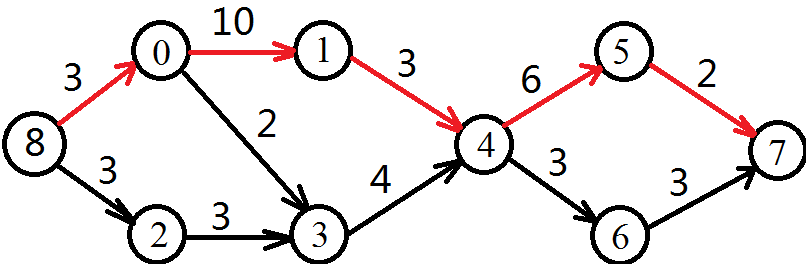我们发现，这一条关键路径一定是一个拓扑排序的子序列。所以我们就可以用拓扑排序计算关键路径了。但问题是拓扑排序有多种结果，怎么才能拿到关键路径 (权重的和最大) 呢？

首先，我们需要计算每个节点的earlyTime (最早开始时间)。终点的earlyTime 就是从起点到终点的最大权重和。
其次，如果我们从终点到起点，进行反向的计算lastTime (最晚开始时间)，如果某一条路径的每一个结点的earlyTime 与lastTime都相等，那么这条路径就是关键路径。

怎么计算每个节点的earlyTime呢？我们可以用递推。
例如：从起点开始，有两条路径可以到达3号点。分别是：8 -> 0 -> 3和8 -> 2 -> 3。我们只需要取这两条路径的权重最大值，那就是8 -> 3的权重。递推关系式：
earlyTime(0) = max[earlyTime(0), earlyTime(8) + weight8_0]
earlyTime(2) = max[earlyTime(2), earlyTime(8) + weight8_2]

earlyTime(3) 需要计算两次：
earlyTime(3) = max[earlyTime(3), earlyTime(0) + weight0_3]
earlyTime(3) = max[earlyTime(3), earlyTime(2) + weight2_3]

用同样的方法就可以计算出终点7的earlyTime，也就是从起点到终点的最大权重和。

在得到从起点到终点的最大权重和之后，我们就可以反向计算了。显然，终点的lastTime 和earlyTime是同一个值。那么其它结点的lastTime的初始值是多少呢？可以简单的赋值为∞ (无穷大，inf)，或者也同样设置为终点的lastTime。

计算结点的lastTime与计算earlyTime类似，也可以用递推。例如一共有两条路径从终点出发，反向走到结点4，分别是7 -> 5 -> 4和7 -> 6 -> 4。递推关系式：
lastTime(5) = min[lastTime(5), lastTime(7) - weight5_7]
lastTime(6) = min[lastTime(6), lastTime(7) - weight6_7]

lastTime(4) 需要计算两次：
lastTime(4) = min[lastTime(4), lastTime(5) - weight4_5]
lastTime(4) = min[lastTime(4), lastTime(6) - weight4_6]
用同样的方法就可以计算出所有结点的lastTime。接着对比一下每个结点的earlyTime和lastTime。如果这两个值相等，则这个结点一定是关键路径上的结点，输出这个结点的编号即可。

总体的代码如下：

#include <stdio.h>
#include <stdlib.h>

int arr; //存放weight的二维数组
int size = 9; //图上的结点数
int inf = 999; //无穷大

//声明结构体:结点
struct Node{
int index; //编号
int inDegree; //入度
int earlyTime = 0; //最早的发生时间，默认为0
int lastTime = inf; //最晚的发生时间，默认为无穷大
struct Node * next; //下一个结点的指针
};

int stack ; //用栈辅助实现拓扑排序，用数组实现栈
int top = 0; //栈的头元素的index

int queue; //一个用数组实现的队列
int tail = 0; //队列的尾

//用一个数组存放首元素。每个首元素后面用指针连接各个结点
struct Node arrNode;

//头插法，得到的结果的次序是反的是得到的结果的次序是反的
{
struct Node * parentNode;
parentNode = &arrNode[parentIndex];

//向操作系统申请空间
struct Node *temp = (struct Node *)(malloc(sizeof(struct Node)));
temp->index = nodeIndex;
temp->next = parentNode->next;

parentNode->next = temp;
arrNode[nodeIndex].inDegree++; //入度 + 1
temp->inDegree = arrNode[nodeIndex].inDegree;
}

//遍历一个结点，和它的所有相连的结点
void traverse(int parentIndex)
{
struct Node * node = &arrNode[parentIndex];
while(node != NULL)
{
printf("%d (%d) --> ", node->index, node->inDegree);
node = node->next;
}
printf("\r\n");
}

int max(int a, int b) //求两个数中的最大值
{
return a > b ? a : b; //三元运算符
}

int min(int a, int b) //求两个数中的最小值
{
return a < b ? a : b; //三元运算符
}

//用二维数组储存图，其实要的仅仅是weight
void initMap()
{
for(int i = 0; i < size; i++)
{
for(int j = 0; j < size; j++)
{
arr[i][j] = 0;
}
}

//对有向图中的边进行赋值
arr = 10; arr = 2; arr = 3; arr = 3; arr = 4; arr = 6;
arr = 3; arr = 2; arr = 3; arr = 3; arr = 3;
}

int TopologicalOrderByStack() //栈辅助实现的拓扑排序
{
int count = 0; //用来计数
int index = 0; //输出的结点的编号
int i = 0; //循环变量

//1、遍历所有结点，寻找入度为0的结点，并把编号存放在stack中
for(i = 0; i < size; i++)
{
struct Node node = arrNode[i];
if(node.inDegree == 0)
{
stack[top] = i; //把结点的编号存放到stack中
top++; //top + 1
}
}

//2、弹出栈中的结点，输出结点编号。同时让该结点的下一级结点的入度-1
//3、循环，直到栈中的结点为0，即top == 0
while(top > 0)
{
top--; //top的位置没有内容，所以要先 - 1
index = stack[top]; //得到存放在stack中的编号
queue[tail] = index; //把编号存放到queue中
tail++; //存放数据后，队列的tail + 1

printf("%d(%d) -> ", index, arrNode[index].earlyTime); //输出编号和earlyTime
count++; //计数 + 1

//从arrNode中获得结点的指针
struct Node * parentNode = &arrNode[index]; //得到数组arrNode中的指定节点
struct Node * node = parentNode->next; //得到arrNode中的指定节点的子节点

//遍历
while(node != NULL) //如果子节点不是NULL就循环
{
int sonIndex = node->index; //子节点的index

//计算子节点的earlyTime
arrNode[sonIndex].earlyTime = max(arrNode[sonIndex].earlyTime, arrNode[index].earlyTime + arr[index][sonIndex]);

//从arrNode中获得结点、结点信息
if(arrNode[sonIndex].inDegree > 0) //子节点的入度 > 0
{
//结点的inDegree - 1
arrNode[sonIndex].inDegree--;

//如果结点的inDegree == 0
if(arrNode[sonIndex].inDegree == 0)
{
//把结点的index (也就是sonIndex) 加入到stack中
stack[top] = sonIndex;
top++;
}
}
node = node->next; //指针指向下一个子节点
}
}

if(count < size) //如果输出的结点数 < 总结点数
{
return 0; //不存在拓扑排序
}
return 1; //存在拓扑排序
}

void releaseResource() //释放资源
{
//同前
}

void showQueue()
{
printf("\r\n显示队列：\r\n");

{
}
printf("\r\n\r\n");
}

void getKeyRoute() //关键路径
{
//最终结点的最晚发生时间就是它的最早发生时间
arrNode[queue[tail - 1]].lastTime = arrNode[queue[tail - 1]].earlyTime;

//从终点往起点开始，反着计算。这种计算方式的时间复杂度为O(N^2)
for(int i = tail - 1; i >= head; i--)
{
for(int j = i; j >= head; j--)
{
if(arr[queue[j]][queue[i]] > 0)
{
//注意，这是一个有向图。所以不能用arr[queue[i]][queue[j]]，
//arr[queue[i]][queue[j]]的值一定是0，用arr[queue[j]][queue[i]]
arrNode[queue[j]].lastTime = min(arrNode[queue[j]].lastTime, arrNode[queue[i]].lastTime - arr[queue[j]][queue[i]]);

//显示每个节点的lastTime
//printf("arrNode[%d].lastTime = %d, arr[queue[j]][queue[i]] = %d\r\n", queue[j], arrNode[queue[j]].lastTime, arr[queue[j]][queue[i]]);
}
}
}
printf("\r\n\r\n显示每个节点和它的lastTime：\r\n");
for(int i = 0; i < size; i++)
{
printf("%d (%d), ", queue[i], arrNode[queue[i]].lastTime);
}

printf("\r\n\r\n关键路径：\r\n");
for(int i = 0; i < size; i++)
{
//如果结点的earlyTime与反向计算得到的lastTime相等，说明是关键路径上的点
//但是，如果有多条关键路径，这里只会傻傻的一起输出，而不是分开输出。
if(arrNode[queue[i]].earlyTime == arrNode[queue[i]].lastTime)
{
printf("%d(%d), ", queue[i], arrNode[queue[i]].lastTime);
}
}
}

int main()
{
initMap(); //初始化地图 (weight)

//初始化
for(int  i = 0; i < size; i++)
{
arrNode[i].index = i;
arrNode[i].inDegree = 0;
arrNode[i].next = NULL;
}

//插入数据

for(int i = 0; i < size; i++) //对每个节点遍历它的相邻节点
{
traverse(i);
}

if(TopologicalOrderByStack())
{
printf("存在拓扑排序\r\n");
}
else
{
printf("不存在拓扑排序\r\n");
}
///完成拓扑排序和计算earlyTime

showQueue(); //显示队列中的内容，这里把队列当初数组看

printf("拓扑排序的尾元素：%d\r\n", queue[tail - 1]);

getKeyRoute(); //计算关键路径

releaseResource(); //释放资源
printf("\r\nHello\r\n");
return 0;
}

运行结果如下：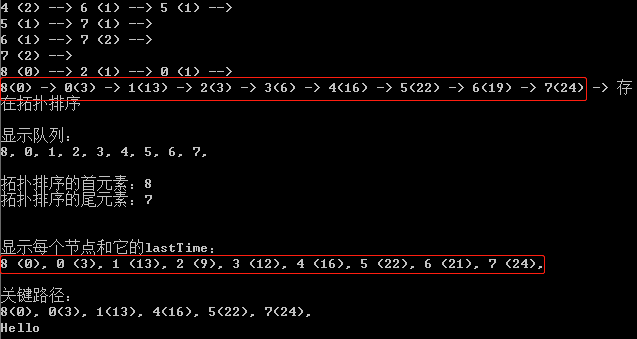很明显，关键路径上的结点的earlyTime与lastTime都相等。如果一个有向图中有多条关键路径，这个代码不会分别显示。只会把所有结点按照拓扑排序中的次序依次显示。
这个算法的思路不难，但是过程却比较复杂，代码也相对比较长。代码中大部分是拓扑排序的内容，真正计算关键路径的代码其实不多。另外，这里使用邻接矩阵的方式储存图的边长 (weight)，需要两层for循环才能最终确定每一个结点的lastTime，所以关键路径算法的时间复杂度为O(N^2)。

关键路径算法是“图”的最后一个扩展算法。它是运筹学的一部分。根据关键路径，可以明确加快哪些步骤可以加速整个工程的进度。

展开全文数据结构 算法
• 图-关键路径算法

千次阅读 2020-08-17 13:49:08
关键路径(CriticalPath) 我们把路径上各个活动所持续时间之和称为路径长度，从源点到汇点具有最大长度的路径叫关键路径，在关键路径上的活动叫做关键活动.。 AOV网和AOE网 算法用到的4个关键变量: **etv ...

关键路径(CriticalPath)

我们把路径上各个活动所持续时间之和称为路径长度，从源点到汇点具有最大长度的路径叫关键路径，在关键路径上的活动叫做关键活动.。

AOV网和AOE网算法用到的4个关键变量:

1. **etv (Earliest time of vertex)：**事件的最早发生时间，即顶点k的最早发生时间
2. **ltv(Latest time of vertex)：**事件最晚发生时间，即顶点k的最晚发生时间
3. **ete(Earliest time of edge)：**活动最早开工时间，即弧k的最早发生时间
4. **lte(Latest time of edge)：**活动最晚开工时间，即弧k的最晚发生时间

etv[k] 与 ltv[k]的计算公式：关键路径算法借助到了改进过的拓扑排序算法，多了一个stack2栈，用来存放倒过来的拓扑序列(由于栈是后进先出的),同时计算生成了etv数组,计算公式如上图。

#include <stdio.h>
#include <stdlib.h>
#define OK 1
#define ERROR 0
#define STATUS int
typedef struct EdgeNode
{
int weight;/*权值*/
struct EdgeNode *next;
}EdgeNode;

typedef struct VertexNode
{
int in; /*入度*/
int data;
EdgeNode *firstEdge;

typedef struct
{
int numVertexes, numEdges;

/*改进的辅助拓扑排序算法*/
/*需要定义几个全局变量*/
int *etc, *ltv;
int *stack2;/*stack2存放拓扑序列的栈*/
int top2; /* top2必须是全局变量，以为在辅助算法和主算法都用到了 */
{
EdgeNode *e;
int i, k, gettop;
int top = 0;
int count = 0;
int	top2 = 0;/*初始化stack2数组的栈顶指针*/
int *stack;

stack = (int *)malloc(G->numVertexes * sizeof(int));
etv = (int *)malloc(G->numVertexes * sizeof(int));/*初始化*/
stack2 = (int *)malloc(G->numVertexes * sizeof(int));/*初始化*/

for(i = 0; i < G->numVertexes; i++)
{
etv[i] = 0;/*每个事件的最早发生时间，都先初始化为0*/

{
stack[++top] = i;/*如果入度为0，入栈*/
}
}

while(top != 0)
{
gettop = stack[top--];
count++;
stack2[++top2] = gettop;/*stack2栈用于保存拓扑序列，后进先出，所以是倒过来的*/

for(e = G->adjList[gettop].firstEdge; e; e = e->next)
{
{
stack[++top] = k;
}

if((etv[gettop] + e->weight) > etv[k])
{
/*gettop就是刚刚弹出的顶点，k就是与gettop相邻的顶点 找一个大的值保存*/
/*v[gettop] ----[weight]----> v[k]*/
/*确定顶点k事件的最早发生时间并且保存以供后续的关键路径算法使用。*/
etv[k] = etv[gettop] + e->weight;
}
}
}

if(count < G->numVertexes)
{
return ERROR;
}else
{
return OK;
}
}

{
EdgeNode *e;
int i, gettop, k, j;
int ete, lte;
TopologicalSort(G);
ltv = (int *)malloc(G->numVertexes * sizeof(int))
for(i = 0; i < G->numVertexes; i++)
{
ltv[i] = etv[G->numVertexes-1];/*所有事件的最晚发生时间都初始化为 拓扑序列最后一个顶点事件的最早发生时间*/
}
/*从后往前开始计算ltv，ltv的计算公式在图中给出*/
while(top2 != 0)
{
gettop = stack2[top2--];
for(e = G->adjList[gettop].firstedge; e; e = e->next)
{
if(ltv[k] - e->weight < ltv[gettop])
{
/* v[gettop] -----[weight]------> v[k] */
itv[gettop] = ltv[k] - e->weight;
}
}
}
for(j = 0; j < G->numVertexes; j++)
{
for(e = G->adjList[j].firstedge; e; e = e->next)
{
ete = etv[j]; /*活动最早开始时间就等于事件最早发生时间*/
lte = ltv[k] - e->weight; /*活动最晚开始时间等于 后继事件最晚发生时间 减去 活动的持续时间(权值)*/
if(ete == lte)
{
/*输出打印*/
}
}
}
}

展开全文数据结构
• 关键路径算法 Java实现

千次阅读 2017-03-09 14:51:45
如果要对一个流程图活的最短时间，就必须分析他们的拓扑关系，并且找到当中最关键的流程， 这个流程的时间就是最短时间 AOE网（Activity On Edge Network）:  * 在一个表示工程的带权有向图中，用顶点表示事件，...

如果要对一个流程图活的最短时间，就必须分析他们的拓扑关系，并且找到当中最关键的流程，

这个流程的时间就是最短时间

AOE网（Activity On Edge Network）:
* 在一个表示工程的带权有向图中，用顶点表示事件，用有向边表示活动，用边上的权值表示活动的持续时间。
* 注：与AOV网不同的是，AOV网是顶点表示活动的网，它值描述活动之间的制约关系，而AOE网是用边表示活动的网，边上的权值表示活动持续的时间。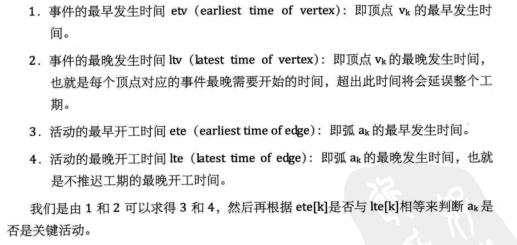结合 图1中的v3,v4理解事件的最早发生时间，v1理解事件的最晚发生时间比较好。

代码中的图

图1：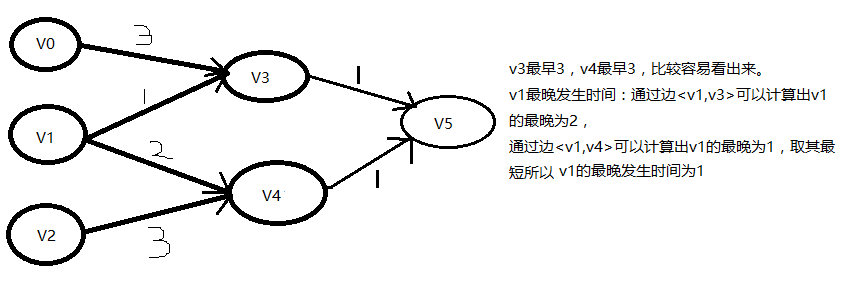图1中事件的最早和最晚发生时间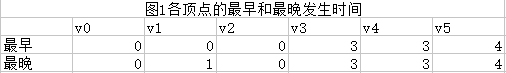图2：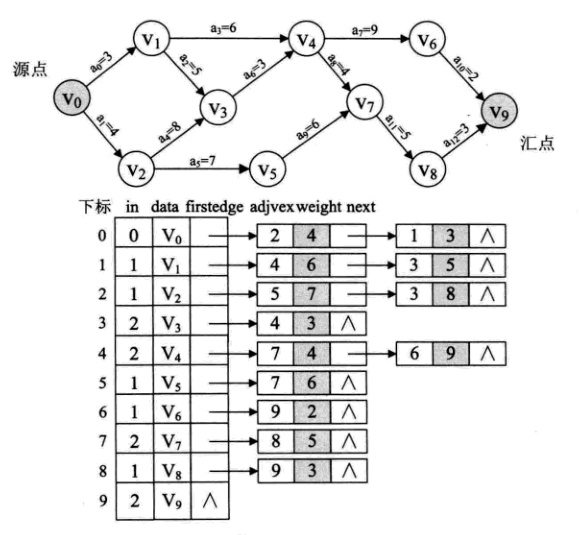图2中事件的最早和最晚发生时间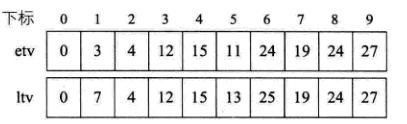代码：

import java.util.ArrayList;
import java.util.List;
import java.util.Stack;

/**
* AOE网（Activity On Edge Network）:<p>
* 在一个表示工程的带权有向图中，用顶点表示事件，用有向边表示活动，用边上的权值表示活动的持续时间。<p>
* 注：与AOV网不同的是，AOV网是顶点表示活动的网，它值描述活动之间的制约关系，而AOE网是
* 用边表示活动的网，边上的权值表示活动持续的时间。
*/
public class CriticalPath {

/**
* 顶点数组
*/
private List<VertexNode> vexList;

/**
* etv:earliest time of vertex 事件的最早发生时间<p>
* ltv:latest time of vertex 事件的最晚发生时间
*/
private int etv[], ltv[];

/**
* 拓扑序列，保存各顶点拓扑排序的顺序
*/
private Stack<Integer> stack2;

/**
* 创建图1（邻接表）
*/
public void createGraph1() {
//v0
VertexNode v0 = new VertexNode(0, 0, null);

EdgeNode v0e0 = new EdgeNode(3, 3, null);
v0.setFirstEdge(v0e0);

//v1
VertexNode v1 = new VertexNode(0, 1, null);

EdgeNode v1e0 = new EdgeNode(3, 1, null);
EdgeNode v1e1 = new EdgeNode(4, 2, null);

v1.setFirstEdge(v1e0);
v1e0.setNext(v1e1);

//v2
VertexNode v2 = new VertexNode(0, 2, null);

EdgeNode v2e0 = new EdgeNode(4, 3, null);
v2.setFirstEdge(v2e0);

//v3
VertexNode v3 = new VertexNode(2, 3, null);

EdgeNode v3e0 = new EdgeNode(5, 1, null);
v3.setFirstEdge(v3e0);

//v4
VertexNode v4 = new VertexNode(2, 4, null);

EdgeNode v4e0 = new EdgeNode(5, 1, null);
v4.setFirstEdge(v4e0);

//v5
VertexNode v5 = new VertexNode(2, 5, null);

vexList = new ArrayList<>();
}

/**
* 创建图2（邻接表）
*/
public void createGraph2() {
//v0
VertexNode v0 = new VertexNode(0, 0, null);

EdgeNode v0e0 = new EdgeNode(2, 4, null);
EdgeNode v0e1 = new EdgeNode(1, 3, null);

v0.setFirstEdge(v0e0);
v0e0.setNext(v0e1);

//v1
VertexNode v1 = new VertexNode(1, 1, null);

EdgeNode v1e0 = new EdgeNode(4, 6, null);
EdgeNode v1e1 = new EdgeNode(3, 5, null);

v1.setFirstEdge(v1e0);
v1e0.setNext(v1e1);

//v2
VertexNode v2 = new VertexNode(1, 2, null);

EdgeNode v2e0 = new EdgeNode(5, 7, null);
EdgeNode v2e1 = new EdgeNode(3, 8, null);

v2.setFirstEdge(v2e0);
v2e0.setNext(v2e1);

//v3
VertexNode v3 = new VertexNode(2, 3, null);

EdgeNode v3e0 = new EdgeNode(4, 3, null);

v3.setFirstEdge(v3e0);

//v4
VertexNode v4 = new VertexNode(2, 4, null);

EdgeNode v4e0 = new EdgeNode(7, 4, null);
EdgeNode v4e1 = new EdgeNode(6, 9, null);

v4.setFirstEdge(v4e0);
v4e0.setNext(v4e1);

//v5
VertexNode v5 = new VertexNode(1, 5, null);

EdgeNode v5e0 = new EdgeNode(7, 6, null);

v5.setFirstEdge(v5e0);

//v6
VertexNode v6 = new VertexNode(1, 6, null);

EdgeNode v6e0 = new EdgeNode(9, 2, null);

v6.setFirstEdge(v6e0);

//v7
VertexNode v7 = new VertexNode(2, 7, null);

EdgeNode v7e0 = new EdgeNode(8, 5, null);

v7.setFirstEdge(v7e0);

//v8
VertexNode v8 = new VertexNode(1, 8, null);

EdgeNode v8e0 = new EdgeNode(9, 3, null);

v8.setFirstEdge(v8e0);

//v9
VertexNode v9 = new VertexNode(2, 9, null);

vexList = new ArrayList<>();
}

/**
* 拓扑排序 用于关键路径计算<p>
*
* 新加了一些代码
*/
public boolean topologicalSort() {
//统计输出顶点数
int count = 0;

//建栈存储入度为0的顶点
Stack<Integer> stack = new Stack<>();

//统计入度数
for (int i = 0;i < vexList.size(); i++) {
vexList.get(i).setIn(0);
}
for (int i = 0;i < vexList.size(); i++) {

EdgeNode edge = vexList.get(i).getFirstEdge();
while (edge != null) {
vex.setIn(vex.getIn() + 1);

edge = edge.getNext();
}
}

//将入度为0 的顶点入栈
for (int i = 0;i < vexList.size(); i++) {
if (vexList.get(i).getIn() == 0) {
stack.push(i);
}
}

//----新加begin---- 初始化
etv = new int[vexList.size()];
stack2 = new Stack<>();
//----新加end----

System.out.print("拓扑序列：");
while (!stack.isEmpty()) {
//栈顶 顶点出栈
int vexIndex = stack.pop();
System.out.print(vexIndex + "  ");

count++;

//----新加 。将弹出的顶点序号压入拓扑序列的栈
stack2.push(vexIndex);

EdgeNode edge = null;
//----循环方式变了一下
for (edge = vexList.get(vexIndex).getFirstEdge(); edge != null; edge = edge.getNext()){

//将此 顶点的入度减一
vex.setIn(vex.getIn() - 1);
//此顶点的入度为零则入栈，以便于下次循环输出
if (vex.getIn() == 0) {
}

//----新加 求各顶点的最早发生时间值。
if (etv[vexIndex] + edge.getWeight() > etv[adjvex]) {
}
}
}

if (count != vexList.size())
return false;
else
return true;
}

/**
* 关键路径
*/
public void criticalPath() {
//求拓扑序列，计算数组etv和stack2的值
boolean success = topologicalSort();
if (!success) {
System.out.println("\n有回路");
return;
}

//声明活动最早发生时间和最迟发生时间
int ete, lte;

//初始化ltv
ltv = new int[vexList.size()];
for (int i = 0; i <vexList.size(); i++)
ltv[i] = etv[vexList.size() - 1];

System.out.print("\n关键路径:\n");
//求顶点的最晚发生时间
while (!stack2.isEmpty()) {
//将拓扑序列出栈
int vexIndex = stack2.pop();

EdgeNode edge = null;
for (edge = vexList.get(vexIndex).getFirstEdge();
edge != null; edge = edge.getNext()) {

//求各顶点最晚发生时间
//已知最早发生时间，才能求最晚发生时间，顺序不能倒过来
//最晚完成时间要按拓扑序列逆推出来
//个人理解：求最晚和最早原理相同，只不过是返回来
if (ltv[adjvex] - edge.getWeight() < ltv[vexIndex]) {
}
}
}

for (int i = 0; i < vexList.size(); i++) {
EdgeNode edge = null;
for (edge = vexList.get(i).getFirstEdge(); edge != null; edge = edge.getNext()) {

//活动最早发生时间，即为边的弧头的最早发生时间
ete = etv[i];
//活动最晚发生时间，即为弧尾的的最晚发生时间减去权值
//相等即为关键路径
if (ete == lte) {
System.out.printf("<v%d,v%d>: %d\n",
}
}
}
}

public static void main(String[] args) {
CriticalPath criticalPath = new CriticalPath();
criticalPath.createGraph1();
//criticalPath.createGraph2();
criticalPath.criticalPath();
}

}

/**
* 边表结点
*
*/
class EdgeNode {
/**
* 邻接点域，存储该顶点对应的下标
*/

/**
* 用于存储权值，对于非网图可以不需要
*/
private int weight;

/**
* 链域，指向下一个邻接点
*/
private EdgeNode next;

public EdgeNode(int adjvex, int weight, EdgeNode next) {
super();
this.weight = weight;
this.next = next;
}

}

}

public int getWeight() {
return weight;
}

public void setWeight(int weight) {
this.weight = weight;
}

public EdgeNode getNext() {
return next;
}

public void setNext(EdgeNode next) {
this.next = next;
}

}

/**
* 顶点表结点
*
*/
class VertexNode {
/**
* 顶点入度
*/
private int in;

/**
* 顶点域，存储顶点信息（下标）
*/
private int data;

/**
* 边表头指针
*/
private EdgeNode firstEdge;

public VertexNode(int in, int data, EdgeNode firstEdge) {
super();
this.in = in;
this.data = data;
this.firstEdge = firstEdge;
}

public int getIn() {
return in;
}

public void setIn(int in) {
this.in = in;
}

public int getData() {
return data;
}

public void setData(int data) {
this.data = data;
}

public EdgeNode getFirstEdge() {
return firstEdge;
}

public void setFirstEdge(EdgeNode firstEdge) {
this.firstEdge = firstEdge;
}

}

结果：

图1：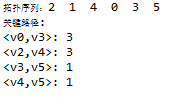图2：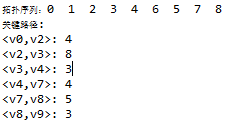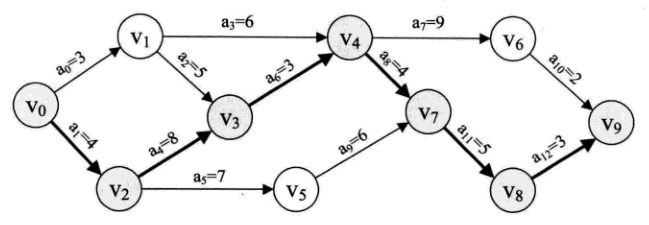展开全文关键路径 算法 Java
• 介绍求关键路经的算法，对于给出的事件结点网络，求出从起点到终点的所有路径，经分析、比较后找出长读最大的路径，从而得出求关键路径算法
• 针对项目管理中关键路径计算这个核心问题，分析了关键路径算法的传统解决方式，深入研究了面向对象方式下解决这个问题的方法，给出了完整、洁净的解决方案，对用面向对象方式解决其他经典问题有帮助。关键路径 面向对象 Java
• 关键路径算法——AOE网

千次阅读 2018-10-15 18:40:58
AOV网——类似于拓扑排序的样子，即用有向图来描述和分析一项工程和计划的实施过程。 AOE网——即在AOV网上加上权重，有向图中以顶点表示事件，有向边代表活动，边上的权重...关键路径算法：   关键路径：AOE...

AOV网——类似于拓扑排序的样子，即用有向图来描述和分析一项工程和计划的实施过程。

AOE网——即在AOV网上加上权重，有向图中以顶点表示事件有向边代表活动，边上的权重代表活动持续的时间。AOE网与AOV网的区别在于：AOE网不仅仅关心整个工程中各个自工程的实施先后顺序，同时也关心整个工程完成的最短时间。

AOE网的特点：只有一个起点和一个终点

关键路径算法：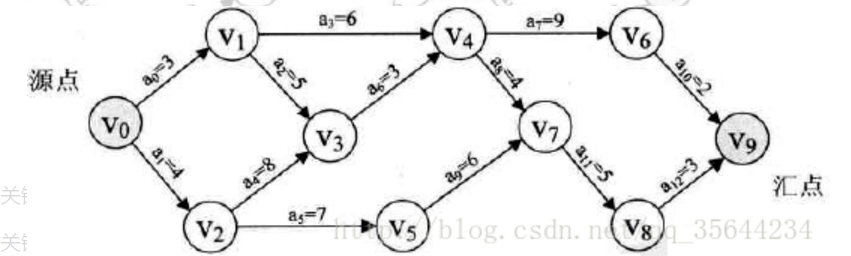关键路径：AOE网中，从起点到终点最长的路径长度（由于要将所有的活动都完成才算是结束，所以只有按照最长时间的活动路径来计算。）长度指的是路径上边的权重和。

关键活动：关键路径上的边。

最早发生时间：从V0到Vi的最长路径的长度。

活动的最早发生时间：e(i)表示活动a(i)最早发生时间，例如从V0到V3，有两条路径，一条8天完成，一条12天完成，但是活动未完成，V3事件开始不了，所以要等到最长的活动结束，V3事件才能开始，即为最早发生时间。

活动最迟发生时间：用 l(i) 表示，不推迟工期的最晚开工时间

最早发生时间和最迟发生时间是不一样的概念。两者区别再后续总结。

关键活动：e(i) = l(i) 的活动 a(i) 称为关键活动。

关键路径的步骤：

以上图为例，最好用表格计算V0——V9事件的关键路径，计算特点：顺序计算！从前往后

 V1 V2 V3 V4 V5 V6 V7 V8 V9 V0开始，最早发生时间e(i) 3 4 12 15 11 24 19 24 27

所以关键路径应为V0——>V2——>V3——>V4——>V7——>V8——>V9，以上数均为各个活动的最早发生时间。

活动最迟发生时间：计算特点：倒序计算！从后往前

 V1 V2 V3 V4 V5 V6 V7 V8 V9 从V9倒推导V0数据 l(i) 9 4 12 15 13 25 19 24 27

在这会发现一个问题，在倒退到V1，V2，V4时，会出现两个数值，选择小的那个数值。

当 e(i) = l(i) 时，a(i)为关键活动。所以我们可以找到关键活动为a(2)，a(3)，a(4)，a(7)，a(8)，a(9)，会发现刚好与上面关键路径相符。

代码暂时忽略，后期补充

展开全文• 算法-数据结构中关键路径算法的实现与应用.rar
• C语言实现图的关键路径算法

千次阅读 2017-08-08 19:11:38
把路径上各个活动所持续的时间之和称为路径长度，从源点到汇点具有最大长度的路径叫关键路径，在关键路径上的活动叫关键活动。 只有缩短关键路径上关键活动的时间才可以减少整个工期长度。 只需要找到所有活动...
• //求AOE网络中的关键路径算法 #include <cstdio> #include <cstring> #define MAXN 100 //顶点个数的最大值 #define MAXM 200 //边数的最大值 struct ArcNode { int to, dur, no; //边的另一个顶点持续时间活动序号 ...
• 关键路径算法.doc关键路径算法.doc关键路径算法.doc关键路径算法.doc关键路径算法.doc关键路径算法.doc关键路径
• c++面向对象方法求图中的关键路径算法，包涵拓扑排序算法关键路径
• 在工程上，我们都很讨厌工程的延期，同时一个工程由分为很多的节点，我们不知道哪些节点决定着这个工程是否会延期，每一个部分有没有可以伸缩的时间，于是，这个用来计划工程的关键路径算法就诞生了。看来，数据结构...数据结构 算法
• 航空产品本身具有高复杂性、协同性要求严格的特征，同时，传统PERT模型已知条件难以充分获取，而且其关键线路求解算法涉及概率论和数理统计知识而难以实现计算机求解。引入灰色理论思想，将传统PERT网络计划已知条件...关键路径 灰色理论
• 采用邻接矩阵表示项目活动网络图需要较多的存储空间，且基于结构化程序设计思想实现网络图和关键路径算法都非常繁琐。采用面向对象的类表示活动，基于动态数组表示活动网络图及活动之间的逻辑关系，并据此开发了基于...
• 关键路径方法（CPM）是一种算法，用于项目管理中以计划一组项目活动。 该程序（cpm）是关键路径算法的实现，该算法可以以最小的总成本和最佳的持续时间来计划一组项目活动。 建筑学 核心组件是cpm.py模块。 该...Python...